Next: Interpretation Up: Finite slit: Diffraction Previous: Finite slit: Diffraction

## Analysis

According to the physical reasoning of the preceding paragraph, we obtain the diffraction pattern of a single slit by substituting d=a/N into Eq. 17 (so that we always have the correct total width a) and then taking the limit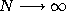(so that we have in the end a single, continuous slit). Recalling that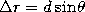, the factor in numerator then becomes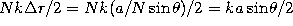. As a similar term appears in the denominator, it is convenient to define the path difference between the top and bottom of the slit (Figure 8)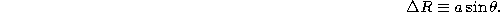With this, the phase factor in the numerator becomes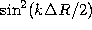. Finally, noting that the argument of the sine function in the denominator is the same but for a missing factor of N, the denominator is just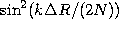.

This leaves for us to take thelimit of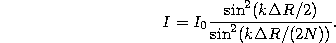As, the argument of the sine function in the denominator becomes quite small, so that we may use the small angle formula,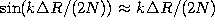. With this, we have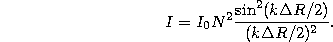From the discussion in Section 3.3.2, we know the intensity at the central maximum to be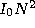. Defining this intensity as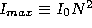, we have our final result for the single slit,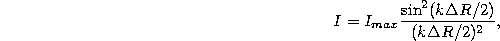where, recall,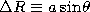.

Tomas Arias
Thu Sep 13 15:26:14 EDT 2001